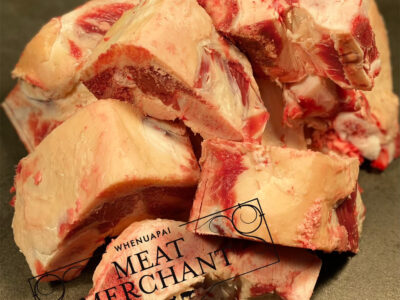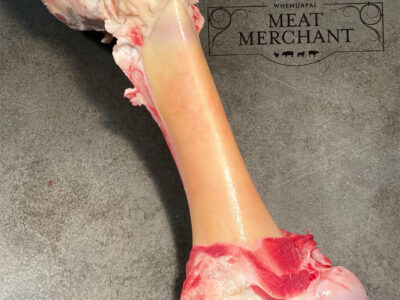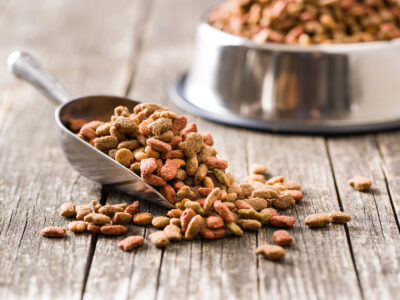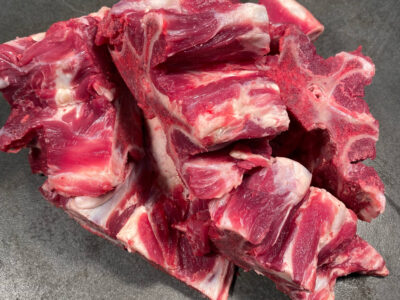Brisket Bones Pet Food\$5.00 jQuery(function(\$){ var price = '5.00'; currency = '\$'; /* \$('[name=quantity]').change(function(){ if (!(this.value < 1)) { var product_total = parseFloat(price * this.value); \$(this).closest('.woocom-list-content').find('.product_total_price .price .woocommerce-Price-amount').html(currency + product_total.toFixed(2)); } }); jQuery( '.variations_form' ).each( function() { jQuery(this).on( 'found_variation', function( event, variation ) { var price = variation.display_price; var quantity = \$(this).find('[name=quantity]').val(); if (!(quantity < 1)) { console.log(price); var product_total = parseFloat(price * quantity); \$(this).closest('.woocom-list-content').find('.product_total_price .price .woocommerce-Price-amount').html(currency + product_total.toFixed(2)); } }); });*/ }); Beef Brisket Bone, soft cartilage and fat. Ideal for young pups or adult dogs, great for cleaning their teeth Brisket Bones quantity ADDCannon Bone Pet Food\$7.00 jQuery(function(\$){ var price = '7.00'; currency = '\$'; /* \$('[name=quantity]').change(function(){ if (!(this.value < 1)) { var product_total = parseFloat(price * this.value); \$(this).closest('.woocom-list-content').find('.product_total_price .price .woocommerce-Price-amount').html(currency + product_total.toFixed(2)); } }); jQuery( '.variations_form' ).each( function() { jQuery(this).on( 'found_variation', function( event, variation ) { var price = variation.display_price; var quantity = \$(this).find('[name=quantity]').val(); if (!(quantity < 1)) { console.log(price); var product_total = parseFloat(price * quantity); \$(this).closest('.woocom-list-content').find('.product_total_price .price .woocommerce-Price-amount').html(currency + product_total.toFixed(2)); } }); });*/ }); Beef Marrow Bone Cut for stockCut in halfWhole Cannon Bone quantity ADDDried Pet Stix Pet Food\$5.00 jQuery(function(\$){ var price = '5.00'; currency = '\$'; /* \$('[name=quantity]').change(function(){ if (!(this.value < 1)) { var product_total = parseFloat(price * this.value); \$(this).closest('.woocom-list-content').find('.product_total_price .price .woocommerce-Price-amount').html(currency + product_total.toFixed(2)); } }); jQuery( '.variations_form' ).each( function() { jQuery(this).on( 'found_variation', function( event, variation ) { var price = variation.display_price; var quantity = \$(this).find('[name=quantity]').val(); if (!(quantity < 1)) { console.log(price); var product_total = parseFloat(price * quantity); \$(this).closest('.woocom-list-content').find('.product_total_price .price .woocommerce-Price-amount').html(currency + product_total.toFixed(2)); } }); });*/ }); Just meat and herbs, the ideal preservative free and gluten free, raw treat. Dried Pet Stix quantity ADDDried Pet Treats Pet Food\$3.00 jQuery(function(\$){ var price = '3.00'; currency = '\$'; /* \$('[name=quantity]').change(function(){ if (!(this.value < 1)) { var product_total = parseFloat(price * this.value); \$(this).closest('.woocom-list-content').find('.product_total_price .price .woocommerce-Price-amount').html(currency + product_total.toFixed(2)); } }); jQuery( '.variations_form' ).each( function() { jQuery(this).on( 'found_variation', function( event, variation ) { var price = variation.display_price; var quantity = \$(this).find('[name=quantity]').val(); if (!(quantity < 1)) { console.log(price); var product_total = parseFloat(price * quantity); \$(this).closest('.woocom-list-content').find('.product_total_price .price .woocommerce-Price-amount').html(currency + product_total.toFixed(2)); } }); });*/ }); Air Dried Pet Treats, from tendons to cartilage to bones. We have a great variety! Dried Pet Treats quantity ADDHeart & Green Tripe Raw Pet Food Pet Food\$9.00 jQuery(function(\$){ var price = '9.00'; currency = '\$'; /* \$('[name=quantity]').change(function(){ if (!(this.value < 1)) { var product_total = parseFloat(price * this.value); \$(this).closest('.woocom-list-content').find('.product_total_price .price .woocommerce-Price-amount').html(currency + product_total.toFixed(2)); } }); jQuery( '.variations_form' ).each( function() { jQuery(this).on( 'found_variation', function( event, variation ) { var price = variation.display_price; var quantity = \$(this).find('[name=quantity]').val(); if (!(quantity < 1)) { console.log(price); var product_total = parseFloat(price * quantity); \$(this).closest('.woocom-list-content').find('.product_total_price .price .woocommerce-Price-amount').html(currency + product_total.toFixed(2)); } }); });*/ }); A blend of Green Tripe and Lamb Heart, an easy meal to feed both dogs and cats to cover their probiotic needs. 1kg Heart & Green Tripe Raw Pet Food quantity ADDLamb Brisket Bones Pet Food\$5.00 jQuery(function(\$){ var price = '5.00'; currency = '\$'; /* \$('[name=quantity]').change(function(){ if (!(this.value < 1)) { var product_total = parseFloat(price * this.value); \$(this).closest('.woocom-list-content').find('.product_total_price .price .woocommerce-Price-amount').html(currency + product_total.toFixed(2)); } }); jQuery( '.variations_form' ).each( function() { jQuery(this).on( 'found_variation', function( event, variation ) { var price = variation.display_price; var quantity = \$(this).find('[name=quantity]').val(); if (!(quantity < 1)) { console.log(price); var product_total = parseFloat(price * quantity); \$(this).closest('.woocom-list-content').find('.product_total_price .price .woocommerce-Price-amount').html(currency + product_total.toFixed(2)); } }); });*/ }); Lamb Brisket Bone. soft cartilage and fat. Ideal for young pups or adult dogs, great for cleaning their teeth Lamb Brisket Bones quantity ADDMeaty Bones Pet Food\$9.00 jQuery(function(\$){ var price = '9.00'; currency = '\$'; /* \$('[name=quantity]').change(function(){ if (!(this.value < 1)) { var product_total = parseFloat(price * this.value); \$(this).closest('.woocom-list-content').find('.product_total_price .price .woocommerce-Price-amount').html(currency + product_total.toFixed(2)); } }); jQuery( '.variations_form' ).each( function() { jQuery(this).on( 'found_variation', function( event, variation ) { var price = variation.display_price; var quantity = \$(this).find('[name=quantity]').val(); if (!(quantity < 1)) { console.log(price); var product_total = parseFloat(price * quantity); \$(this).closest('.woocom-list-content').find('.product_total_price .price .woocommerce-Price-amount').html(currency + product_total.toFixed(2)); } }); });*/ }); Meaty Beef Spine Bone (Chuck Bone) Ideal for stocks & broths Meaty Bones quantity ADD